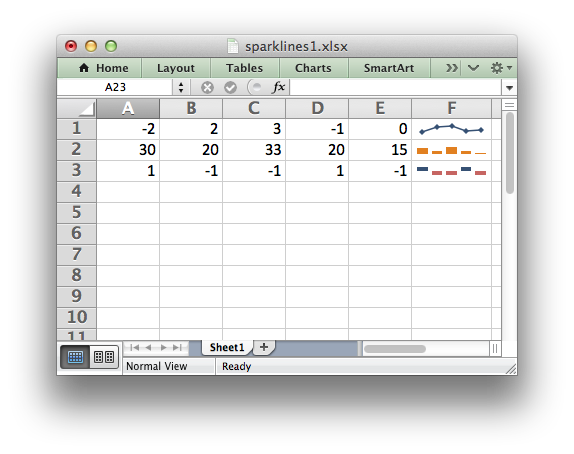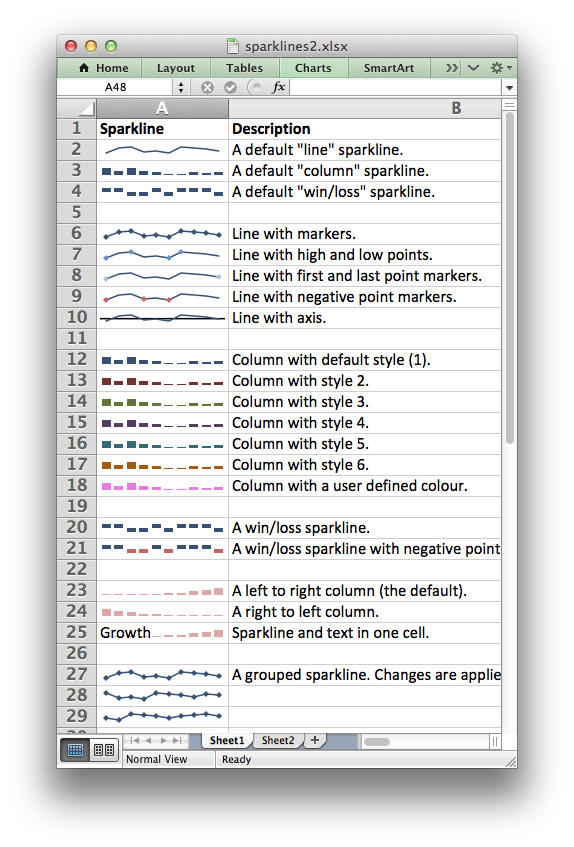# Working with Sparklines#

Sparklines are a feature of Excel 2010+ which allows you to add small charts to worksheet cells. These are useful for showing visual trends in data in a compact format.Sparklines were invented by Edward Tufte: https://en.wikipedia.org/wiki/Sparklines

The `add_sparkline()` worksheet method is used to add sparklines to a cell or a range of cells:

```worksheet.add_sparkline(0, 5, {'range': 'Sheet1!A1:E1'})
```

Both row-column and A1 style notation are supported. See Working with Cell Notation for more details.

The parameters to `add_sparkline()` must be passed in a dictionary. The main sparkline parameters are:

 range (required) type style markers negative_points axis reverse

Other, less commonly used parameters are:

 location high_point low_point first_point last_point max min empty_cells show_hidden date_axis weight series_color negative_color markers_color first_color last_color high_color low_colorThese parameters are explained in the sections below.

Note

Sparklines are a feature of Excel 2010+ only. You can write them to an XLSX file that can be read by Excel 2007 but they won’t be displayed.

## range#

The `range` specifier is the only non-optional parameter.

It specifies the cell data range that the sparkline will plot:

```worksheet.add_sparkline('F1', {'range': 'A1:E1'})
```

The `range` should be a 2D array. (For 3D arrays of cells see “Grouped Sparklines” below).

If `range` is not on the same worksheet you can specify its location using the usual Excel notation:

```worksheet.add_sparkline('F1', {'range': 'Sheet2!A1:E1'})
```

If the worksheet contains spaces or special characters you should quote the worksheet name in the same way that Excel does:

```worksheet.add_sparkline('F1', {'range': "'Monthly Data'!A1:E1"})
```

## type#

Specifies the type of sparkline. There are 3 available sparkline types:

```line (default)
column
win_loss
```

For example:

```worksheet.add_sparkline('F2', {'range': 'A2:E2',
'type': 'column'})
```

## style#

Excel provides 36 built-in Sparkline styles in 6 groups of 6. The `style` parameter can be used to replicate these and should be a corresponding number from 1 .. 36:

```worksheet.add_sparkline('F2', {'range': 'A2:E2',
'type': 'column',
'style': 12})
```

The style number starts in the top left of the style grid and runs left to right. The default style is 1. It is possible to override color elements of the sparklines using the `_color` parameters below.

## markers#

Turn on the markers for `line` style sparklines:

```worksheet.add_sparkline('A6', {'range': 'Sheet2!A1:J1',
'markers': True})
```

Markers aren’t shown in Excel for `column` and `win_loss` sparklines.

## negative_points#

Highlight negative values in a sparkline range. This is usually required with `win_loss` sparklines:

```worksheet.add_sparkline('A9', {'range': 'Sheet2!A1:J1',
'negative_points': True})
```

## axis#

Display a horizontal axis in the sparkline:

```worksheet.add_sparkline('A10', {'range': 'Sheet2!A1:J1',
'axis': True})
```

## reverse#

Plot the data from right-to-left instead of the default left-to-right:

```worksheet.add_sparkline('A24', {'range': 'Sheet2!A4:J4',
'type': 'column',
'style': 20,
'reverse': True})
```

## weight#

Adjust the default line weight (thickness) for `line` style sparklines:

```worksheet.add_sparkline('F2', {'range': 'A2:E2',
'weight': 0.25})
```

The weight value should be one of the following values allowed by Excel:

```0.25, 0.5, 0.75, 1, 1.25, 2.25, 3, 4.25, 6
```

## high_point, low_point, first_point, last_point#

Highlight points in a sparkline range:

```worksheet.add_sparkline('A7', {'range': 'Sheet2!A1:J1',
'high_point': True,
'low_point': True,
'first_point': True})
```

## max, min#

Specify the maximum and minimum vertical axis values:

```worksheet.add_sparkline('F1', {'range': 'A1:E1',
'max': 0.5,
'min': -0.5})
```

As a special case you can set the maximum and minimum to be for a group of sparklines rather than one:

```'max': 'group'
```

See “Grouped Sparklines” below.

## empty_cells#

Define how empty cells are handled in a sparkline:

```worksheet.add_sparkline('F1', {'range': 'A1:E1',
'empty_cells': 'zero'})
```

The available options are:

• `gaps`: show empty cells as gaps (the default).

• `zero`: plot empty cells as 0.

• `connect`: Connect points with a line (“line” type sparklines only).

## show_hidden#

Plot data in hidden rows and columns:

```worksheet.add_sparkline('F3', {'range': 'A3:E3',
'show_hidden': True})
```

Note, this option is off by default.

## date_axis#

Specify an alternative date axis for the sparkline. This is useful if the data being plotted isn’t at fixed width intervals:

```worksheet.add_sparkline('F3', {'range': 'A3:E3',
'date_axis': 'A4:E4'})
```

The number of cells in the date range should correspond to the number of cells in the data range.

## series_color#

It is possible to override the color of a sparkline style using the following parameters:

```series_color
negative_color
markers_color
first_color
last_color
high_color
low_color
```

The color should be specified as a HTML style `#rrggbb` hex value:

```worksheet.add_sparkline('A18', {'range': 'Sheet2!A2:J2',
'type': 'column',
'series_color': '#E965E0'})
```

## location#

By default the sparkline location is specified by `row` and `col` in `add_sparkline()`. However, for grouped sparklines it is necessary to specify more than one cell location. The `location` parameter is used to specify a list of cells. See “Grouped Sparklines” below.

## Grouped Sparklines#

The `add_sparkline()` worksheet method can be used multiple times to write as many sparklines as are required in a worksheet.

However, it is sometimes necessary to group contiguous sparklines so that changes that are applied to one are applied to all. In Excel this is achieved by selecting a 3D range of cells for the data `range` and a 2D range of cells for the `location`.

In XlsxWriter, you can simulate this by passing an array refs of values to `location` and `range`:

```worksheet.add_sparkline('A27', {'location': ['A27',   'A28',   'A29'],
'range':    ['A5:J5', 'A6:J6', 'A7:J7']})
```